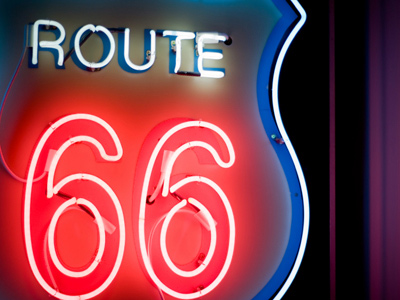The number 66 is closer to 100 than it is to 0.

# Rounding Numbers 2 (Easy)

Welcome to the second of our Easy quizzes on Rounding Numbers. This is all about making approximations which make your estimated answers much closer to the real answer.

Here’s an example:

If you want to know what 48 x 12 is then you might round the numbers to 50 x 10. This makes your estimated answer 500. The real answer should be a similar amount. In fact, 48 x 12 = 576.

To round numbers just ask yourself which ten, hundred or thousand they are nearest to. But don’t forget the rule: 5s, 50s and 500s are always rounded UP not DOWN.

Okay then, are you ready? Let’s begin the quiz. See if you can get all ten questions right.

1.
What is 18,583 rounded to the nearest 1,000?
18,000
18,600
19,000
18,500
583 is closer to 1,000 than 0, so you round upwards
2.
What is 11,345 rounded to the nearest 10?
11,300
11,340
11,400
11,350
Although 5 is equidistant from 0 and 10, the rule means that you round upwards
3.
What is 10,082 rounded to the nearest 10?
10,090
10,100
10,080
10,000
2 is closer to 0 than to 10, so you round downwards
4.
What is 18,425 rounded to the nearest 10?
18,500
18,420
18,400
18,430
Although 5 is equidistant from 0 and 10, the rule means that you round upwards
5.
What is 256 rounded to the nearest 100?
200
300
250
260
56 is closer to 100 than to 0, so you round upwards
6.
What is 26,500 rounded to the nearest 1,000?
26,000
27,000
26,500
26,600
Although 500 is equidistant from 0 and 1,000, the rule means that you round upwards
7.
What is 47 rounded to the nearest 10?
40
45
50
10
7 is closer to 10 than 0, so you round upwards
8.
What is 13,751 rounded to the nearest 100?
13,800
13,750
13,700
14,000
51 is closer to 100 than to 0, so you round upwards
9.
What is 3,498 rounded to the nearest 1,000?
3,000
3,500
4,000
3,400
498 is closer to 0 than to 1,000, so you round downwards
10.
What is 28,486 rounded to the nearest 10?
28,500
28,480
28,400
28,490
6 is closer to 10 than to 0, so you round upwards
Author:  Frank Evans